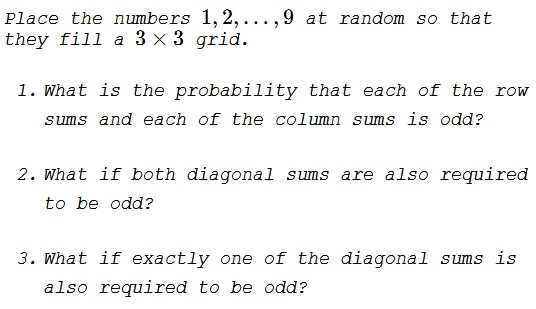# Dropping Numbers into a 3x3 Square

### Problem1) $\displaystyle \frac{1}{14},$ 2) $\displaystyle \frac{1}{126},$ 3) $\displaystyle \frac{2}{63}.$

### Solution 1

1. In order for the sum of three numbers to be odd, they must all be odd or exactly one number is odd. There are five odd numbers available, so one row must have three odd numbers and the other two rows have exactly one odd number with the analogous result for columns.

Once we choose a row with three odd numbers and a column with three odd numbers, we have used up all five available odd numbers. There are $3\times 3 = 9$ ways of choosing the row and column, $5!$ ways of determining where the odd numbers are, and $4!$ ways of determining where the even numbers are. This gives a total of $9\times 5!\times 4!$ matrices with the desired property.

There are a total of $9!$ matrices with entries from $1, 2, \ldots, 9,$ so the probability we seek is

$\displaystyle \frac{9\times 5!\times 4!}{9!}=\frac{1}{14}.$

2. If there is a column and a row with three odd numbers each, there is no way to place three odd numbers on a diagonal. In any event, there is a unique odd number that is shared by the chosen row and column (of the three odd numbers each). For a diagonal sum to be odd this number needs to be on the diagonal. Only in one case out of nine both the diagonal sums are odd; this is when the common odd element is at the center of the matrix. The probability of this is

$\displaystyle \frac{5!\times 4!}{9!}=\frac{1}{126}.$

3. If the selected point is in one of the corners matrix then the corresponding diagonal's sum is odd while that of the other is even. There are four corners and the probability of such an event is

$\displaystyle \frac{4\times 5!\times 4!}{9!}=\frac{4}{126}=\frac{2}{63}.$

### Solution 2

We have $4$ even and $5$ odd numbers. The parity combinations of three numbers that result in an odd sum are $\{O,E,E\}$ and $\{O,O,O\}$.

1. The only possibility is that two rows have two even numbers each and the third row has no even number. Same logic applies to the columns. Thus, we have choose the two rows and the two columns. This can be done in $3\times 3=9$ ways. The two row and two columns set the positions for the evens. The remaining positions are for the odds. Thus, there are $4!$ ways of permuting the evens and $5!$ of permuting the odds. The total number of arbitrary permutations is $9!$. Thus, the probability is

$\displaystyle P_1=\frac{9\cdot 4!\cdot 5!}{9!}=\frac{1}{14}.$

2. For one diagonal to have odd sum, two of the four even positions in the preceding part need to have the same row and column number. If those positions are $(i,i)$ and $(j,j)$, the remaining evens have to occupy $(i,j)$ and $(j,i)$ to satisfy the conditions in part 1. Now the only way the other diagonal sums to an odd number is if $(i,j)$ and $(j,i)$ fall on the other diagonal and $(i,i)$, $(j,j)$ don't fall on that diagonal. Thus, $\{i,j\}=\{1,3\}$. The $9$ possible ways of choosing the even positions drops to $1$ and the probability shrinks by a factor of $9$ to

$\displaystyle P_2=\frac{1}{9\cdot 14}=\frac{1}{126}.$

3. For the main diagonal to have odd sum, we place the two evens at $(i,i)$ and $(j,j)$ as in part 2. However, to keep the sum of the other diagonal to even, $(i,j)$ and $(j,i)$ need to be off that diagonal. Thus, $\{i,j\}=\{1,2\}~\text{or}~\{2,3\}$. Thus, now instead of $9$ ways of choosing the positions of evens in part 1, we drop to $2$ ways of choosing the positions for evens, and the probability drops by a factor of $9/2$. However, we could as well have chosen the second diagonal to give an odd sum instead of the first. This doubles the probability. Thus,

$\displaystyle P_3=\frac{2}{\frac{9}{2}\cdot 14}=\frac{2}{63}.$

### Acknowledgment

This is a slightly expanded version of problem CC246 (Contest Corner, Crux Mathematicorum, Vol. 43(10), December 2017.) The solution is by Missouri State Problem Solving Group. Solution 2 is by Amit Itagi.